American Journal of Theoretical and Applied Statistics
Volume 5, Issue 4, July 2016, Pages: 219-224

Estimation of Change Point in Poisson Random Variables Using the Maximum Likelihood Method

Shalyne Nyambura1, Simon Mundia2, Anthony Waititu1

1Department of Statistics and Actuarial Sciences, Jomo Kenyatta University of Agriculture and Technology, Nairobi, Kenya

2Department of Statistics and Actuarial Sciences, Dedan Kimathi University of Technology, Nyeri, Kenya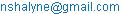(S. Nyambura)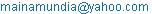(S. Mundia)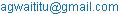(A. Waititu)

Shalyne Nyambura, Simon Mundia, Anthony Waititu. Estimation of Change Point in Poisson Random Variables Using the Maximum Likelihood Method. American Journal of Theoretical and Applied Statistics. Vol. 5, No. 4, 2016, pp. 219-224. doi: 10.11648/j.ajtas.20160504.18

Received: May 27, 2016; Accepted: June 18, 2016; Published: July 11, 2016

Abstract: The point at which a process undergoes a significant shift from its usual course is known as change point. Change point analysis entails testing for the presence of change in a given process, and the location of a single or multiple change points. This study presents a maximum likelihood estimate of a single change point in a sequence of independent and identically distributed Poisson random variables which are dependent on some covariates. A Poisson regression model is used to estimate the mean parameter and the likelihood function. A likelihood ratio test is conducted to check whether change exists with critical values of the test being obtained as in Gombay and Horvath . The procedure is validated for simulated data for cases when there is no change and when there is a predefined change point with special application to incidence of road accidents in Kenya.

Keywords: Change Point, Poisson Regression, Maximum Likelihood Estimation, Likelihood Ratio Test

Contents

1. Introduction

Change is a usual aspect of everyday life. It can be noted perhaps in the socio-economic status of a low income earner who recently won a substantial sum of money in a lottery. It could be noted in the behavior of a young girl who is at the onset of adolescence. It could even be in the health status of an elderly man who has begun daily physical exercises at the local gymnasium. In other words, change is something one can easily relate to. However, change often goes unnoticed and most of the time, only the effects of change are noted at a much later time following its occurrence.

From a statistical viewpoint, the realizations of any scientific process usually vary considerably over a defined threshold, within which the process is said to be in its "usual state". Nevertheless, these realizations may exceed an acceptable threshold or their distributions may change at one point or at multiple points.

If the exact instance when change occurred were known, then perhaps one could be a step closer to establishing the causes that could be attributed to it. This could help in taking appropriate measures to either reinforce these changes or more so to avoid them if they had adverse effects. For instance in medical science, the treatment and management of cancer has posed a great challenge in the current and past century even with the advancement of medical technology. This is mainly attributed to the fact that the success of cancer treatment is to a large extent dependent on the stage of development at which the cancerous growths were detected, Farber , Cooper . However, most cancer patients do not realize that they have the disease until it is in advanced stages of development when the symptoms are obvious. This may be attributed to the relatively long latency period between exposure to carcinogens and the transformation of normal body cells to cancerous cells, Cooper . The earlier the detection and onset of treatment of cancer the more manageable it is.

Historically control charts, such as the CUSUM charts and Shewhart charts, were the most popular monitoring tools used to detect deviations in various processes. These charts were developed in the 1950’s for industrial quality control. They signaled that a process was out of control once measurements departed significantly from some predefined benchmark values.

However, it was noted with concern that the point at which a control chart gave an out-of-control signal was not the actual point of change, but rather a belated point.

Change point analysis was initially introduced in the quality control context as an improvement on the method of control charting. With CPA, it was possible not only to detect the presence of change in various processes, but also to locate the point of change. However, the two methods-control charting and CPA, are often used to complement each other, Amiri .

Over the years CPA has developed into a fundamental problem with applications in various fields including but not limited to; dose-response surveys, credit scoring, identifying structural breaks, studying major drifts in weather patterns and earthquake patterns.

The study of change points has evolved in time from the detection and estimation of a single change point, to that of multiple change points in a system. It has been applied over time to offline and online data sequences from various distributions. Different approaches to estimation of change points have been employed, the most common of which are Bayesian and likelihood-based approaches. The following sections 2 and 3 give a summary of the methodology applied and results obtained.

2. Methodology

2.1. Generalized Linear Models

Generalized Linear Models, as in Nelder  are a class of linear models that provide an avenue to model a response variable against several predictor variables without requiring a linear relationship between individual predictors and the response variable. GLM’s have three main components: a random component which specifies the probability distribution of the response variable; a systematic component and a link function.

GLM’s are based on the assumption that the distribution functions of the response variables belong to the exponential family of distributions with a given mean that specifies the form of the link function, Lee (2007). Particularly, a random variable Y is said to belong to the exponential family of distributions if its probability distribution can be expressed in the form: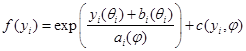(1)

For some constants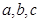and scale and location parameters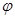and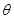respectively

Some models that belong to the class of generalized linear models are: the simple linear regression model, the logistic regression models, the log-linear models and the Poisson regression model.

The Poisson regression model has the natural logarithm as the canonical link function. The link function allows the response variable to relate to the explanatory variables through a set of regression coefficients. This model rides on the basic assumption that the probability distribution of the response variable under consideration is the Poisson distribution. Particularly, a random variable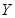is said to follow a Poisson distribution with mean parameterif it takes integer values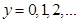with probability function: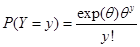(2)

It can be shown that the Poisson variablebelongs to the exponential family of distributions.

In this model the natural logarithm of the expected value of the response variable, which is the Poisson mean parameteris expressed as a linear combination of the predictor variables. The Poisson regression model with log link takes the form: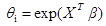(3)

This is equivalent to the linear form expressed as: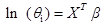(4)

Where;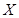is a vector of explanatory variables,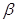is a vector of regression coefficients,is the Poisson mean parameter.

2.2. The Poisson Regression Change Point Model

The general concept behind detection of a single change point is binary splitting. This entails the partitioning of a sequence of realizations of a random variable into two sub-sequences; those cases whose values fall below some value, say k, and those whose values are above this value. The constant k is known as the change point. The change-point is chosen such that it maximizes the distinction between the two sub-sequences, Boudjellaba . Similarly for a multiple change point problem, this binary splitting algorithm is applied recursively to each sub-sequence to obtain further change points, until the change points are exhausted. Several methods have been studied to estimate the locations of change points so far, including Bayesian and frequentist approaches, Chen and Gupta . In this study the binary splitting algorithm is performed for the single change point case with respect to Poisson data sequences and maximum likelihood approach used to estimate the change point.

Consider a sequence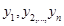from the distribution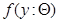. Let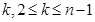be an arbitrary point that partitions the sequence so that the first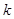observations follow the distribution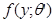while the rest of the observations after point, have the distribution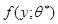for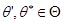. In other words, the two sub-sequences have a common distributional form but the parameters are different, so that the firstobservations follow a distribution with the parameter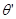, while observations after the point k have a distribution with the parameter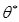. This pointwhere there is a shift in the form of the distribution of the sequence, if it exists, is the change point. However, if there is no significant change the distribution of the entire sequence has a common parameterTo check whether a change exists in this sequence, a likelihood ratio test with a null hypothesis of no change is performed. Mathematically the test hypotheses are written as: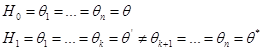(5)

Under the null hypothesis the likelihood function is: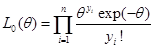(6)

Where the MLE of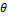is obtained through Poisson regression as;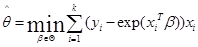(7)

Under the alternative hypothesis, the likelihood function takes the form: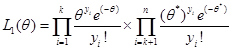(8)

Where the MLE’s of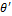and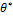are obtained through Poisson regression as: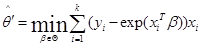(9)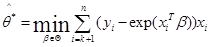(10)

The likelihood ratio for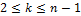is obtained as: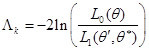(11)

The likelihood ratio test statistic that is used in this study is;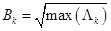(12)

The change pointis estimated such that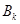is maximized. The null hypothesis is rejected for large values of, that is if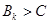where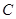is a constant that is determined by the size of the test, the sample size and the null distribution of this test statistic. The reader is referred to Gombay and Horvath  for a detailed illustration of the asymptotic distribution and the asymptotic critical values for the statistic.

Various sample sizes and dimensions were considered and critical values obtained for each sample size at three different levels of the test. See tables of critical values in the appendix.

3. Results and Discussions

3.1. Simulation Study

Simulated observations for the response variable and two independent variables were random numbers generated using the R statistical software as follows: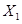, taken to represent the age of the driver, was obtained from a truncated Normal distribution with mean 35 and standard deviation of 10, confined within the integers 18 and 60.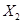, taken to represent the type of vehicle, was obtained from the Bernoulli distribution with parameter 0.6. Particularly it assumed the value 1 for a PSV and 0 otherwise., taken to represent the total annual accident count, was obtained from the Poisson distribution with mean parameter, obtained by fixing the regression coefficients as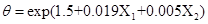for a case of no change;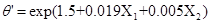and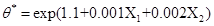for a case when there is a change. This was executed iteratively for three chosen change points,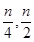and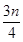for a sample size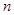and for every value ofa single value ofwas generated.

The model for the Poisson change point described in section 2 was fitted to the simulated data for different sample sizes. The procedure was repeated several times and the test statistic values stored for each value of.

Graphs of the test statistic were plotted and their maximum values compared to the tabulated critical values. For the case of no change with a sample size of 200, it was found that the maximum value of the LRT statistic 4.465 was below each of the critical values for test sizes (see table A1 in the appendix). Therefore the null hypothesis was not rejected; as such, the method correctly showed that there was no change.

Figure 1 shows the result of the LRT for a case of no change with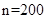. The red line marks the maximum value of the test statistic while the green line marks the critical value at 5% level.

For the case of a preset change point with a sample size of 200, it was found that the maximum value of the LRT statistic 6.45632 was above each of the critical values for all values of alpha (see table A1 in the appendix). Therefore the null hypothesis was rejected; as such, the method correctly showed that there was a change.

Figure 2 shows the results of the LRT for a change at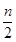when. The redline marks the maximum value of the test statistic whereas the green line marks the critical value at 5% level.

Histograms of change points for the case of a change were plotted to investigate the power of the Likelihood Ratio test. It was noted as expected that majority of change points for different sample sizes lay around the preset change points. The power of the LRT was obtained as a ratio of the number of times the test yielded the correct results for the change points estimates to the total number of iterations. The reader is referred to Mundia and Waititu  for further reading on the power of the LRT for a single change point. The results are summarized in table 1 for some preset change points at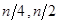and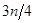for a sample size= 200.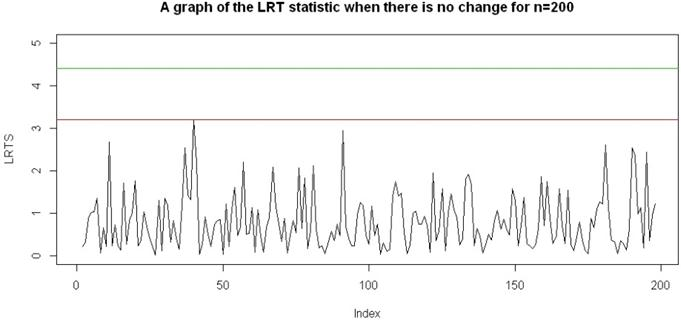Figure 1. The LRT for a case of no change when n=200.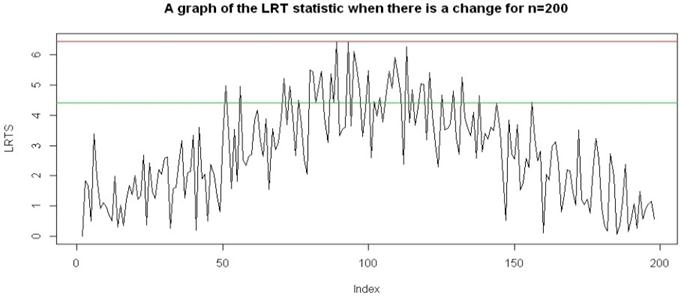Figure 2. The LRT for a change atwhen n=200.

Table 1. Power of test for three preset change points.

 Change Point n/4 n/2 n/3 Number of correct results 100 175 140 Number of iterations 200 200 200 Power of Test 0.50 0.875 0.70

The test was found to be most powerful when the change point was set at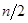. Moreover it was noted that the test was more powerful atcompared to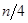even when the two points are in the same relative position in respect of the end points. Thus the distribution of change points is asymmetrical.

3.2. Model Application to Real Data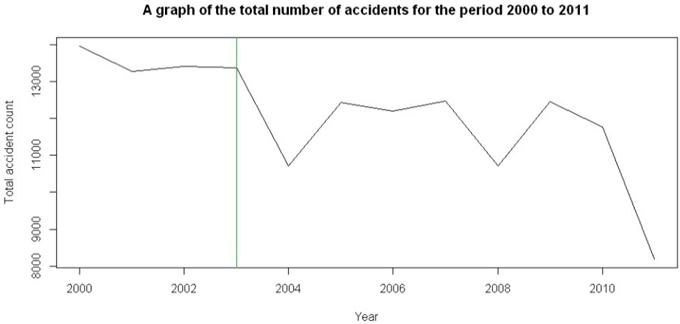Figure 3. A line graph of the real data for the years 2000-2011.

Secondary data on road accidents in Kenya were obtained from the Ministry of Transport, Traffic department for the period 2000-2011. The Poisson response variable Y, was the total annual accident count, presumed to be influenced by four major causes: X1-human errors, X2-non-human errors, X3-bad weather and X4-vehicle and road defects. A line graph, as in figure 3, of the real data for the years 2000 to 2011 reveals that there was a marked decrease in the total number of accidents in the year 2004. Moreover, the annual number of accidents was lower for the block of years 2004-2011 compared to the block of years 2000-2003. This indicates that there was a change at some point during the period under consideration.

3.2.1. Model Selection

Hierarchical Poisson regression models were fitted to the real data and the AIC alongside the deviance statistic used to choose the model that best fit the data. Hierarchical models such as those used in Syamsunder and Naikan  are useful in evaluating the effects of various covariates on the response variable.

The full model included all four independent variables to take the form: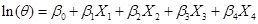(13)

Where;=mean annual accident count.

Three reduced models were obtained by leaving out some of the independent variables. The results obtained for the four models with regard to AIC, the deviance and the corresponding degrees of freedom are summarized in table 2.

Table 2. Analysis of deviance table.

 Model Model variables AIC Deviance df 1 X1, X2, X3, X4 160.47 15.735 7 2 X1, X2, X3, 163.11 20.366 8 3 X1, X2 199.04 58.3 9 4 X1, X3 169.23 28.488 9

With regard to the AIC values, Model 1 was the best choice since it had the smallest AIC.

A chi-square test for change in deviance was constructed at 5% level to compare each of the smaller models against the full model. The hypotheses tested were of the form:

H0: Reduced model is better

H1: Saturated model is better

The p-values for models 2, 3 and were;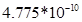, 0.0314 and 0.00174 respectively. The null hypothesis was rejected for all three models since their p-values were lower than 5%. Therefore the most appropriate regression model was Model 1.

3.2.2. Model Fitting

A graph of the LRT statistic for the real data as shown in ﬁgure 5 attained a maximum at the year 2003. This maximum value (3.4271) as marked by the red line exceeded the critical value at 5% level (see table A2 in the appendix) as marked by the green line. This revealed that a signiﬁcant change was present; hence the null hypothesis of no change was rejected. It was concluded that there was a change in the distribution of the two sub-sequences before and after the year 2004 as marked by the vertical line at year 2003. This backed up the ﬁndings from the line graph in ﬁgure 3, that there was a change in the year 2003.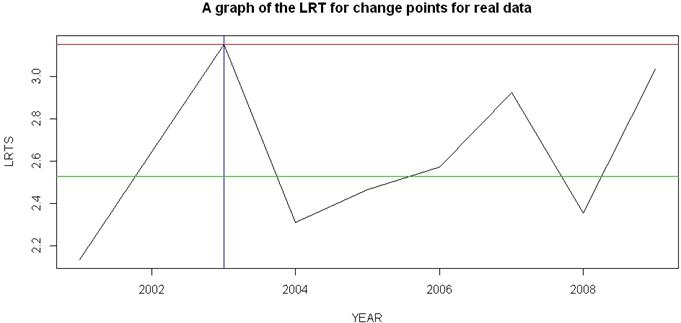Figure 4. The LRT for change for the real data.

4. Conclusions, Limitations and Recommendations

It was found that there was a significant change in the annual number accidents on Kenyan roads in the year 2003 evidenced by the marked drop in accident counts at the end of year 2004. This could be attributed to the enforcement of stringent traffic rules in the year 2003. The famous "Michuki rules" were imposed on the transport sector toward the end of the year 2003 and early 2004 by the then Minister for Transport, the late Hon. John Michuki. However as time passed, these rules were flouted especially by motorists in the public service sector, and the accident rate increased consequently. Since these rules had a marked positive contribution toward the efforts to curb road carnage they should be reinforced by the Ministry of transport.

This study focused on the estimation of a single change point in a sequence of i.i.d. random variables using the maximum likelihood approach. A Poisson regression model was fitted to the data with the necessary assumption that the sequence of variables was derived from a Poisson distribution with equal mean and variance. However, as the case may be in some datasets, the variance may be lower than or exceed the mean, which would rule out the applicability of a Poisson regression model. In such cases, the negative binomial regression model for instance, may be considered. More suggestions on how to deal with non-homogeneous Poisson processes may be found in Chang .

A major drawback faced in this study was dealing with large data values that are problematic under the Poisson model. This problem could possibly be resolved by considering a non-parametric approach. Moreover, an alternative method of estimation to the MLE such as the CUSUM procedure or Bayesian analysis as in Carlin  may be considered. Further, there is a need for improved documentation of data on road accident in Kenya especially with regard to the variables affecting these events.

Appendix: Tables of Critical Values

Table A1. Table of critical values for the simulated data.

 Sample size Test size Critical Values 12 0.01 4.062015 0.05 3.524803 0.10 3.246775 50 0.01 4.28215 0.05 3.783263 0.10 3.531413 100 0.01 4.351433 0.05 3.864997 0.10 3.621652 200 0.01 4.407084 0.05 3.930433 0.10 3.693768

Table A2. Table of critical values for the actual data.

 Sample size Test size Critical Values 12 0.01 2.527259 0.05 2.497973 0.10 2.463733 50 0.01 3.074956 0.05 2.92367 0.10 2.796207 100 0.01 4.270939 0.05 3.712869 0.10 3.41866 200 0.01 4.57571 0.05 4.055173 0.10 3.784107

References

1. Amiri, A. and Allahyari, S. (2011), Change point estimation methods for control chart post-signal diagnostics: A literature review. Quality Reliable Engineering International, 28 (7): 673–685.
2. Boudjellaba, H., MacGibbon, B., and Sawyer, P. (2001), On exact inference for change in a Poisson sequence. Communications in Statistics - Theory and Methods, 30 (3): 407–434.
3. Carlin, B. P., Gelfand, A. E., and Smith, A. F. M. (1992), Hierarchical Bayesian analysis of change point problems, Applied Statistics, 41 (2): 389.
4. Chang, Y. P. (2001). Estimation of parameters for non homogeneous Poisson process: Software reliability with change-point model. Communications in Statistics-Simulation and Computation, 30 (3): 623–635.
5. Chen, J. and Gupta, A. K. (2012), Parametric Statistical Change Point Analysis, Birkhauser Boston.
6. Cooper, G. M. and Hausman, R. E. (2014), The cell: A molecular approach. The Quarterly Review of Biology, 89 (4): 399–399.
7. Cooper, J. A. (2004). Biomedical research, Academic Medicine, 79 (7): 710.
8. Farber, E. (1988), Cancer development and its natural history: A cancer prevention perspective. Cancer, 62 (S1): 1676–1679.
9. Gombay, E. and Horvath, L. (1996), On the rate of approximations for maximum likelihood tests in change-point models, Journal of Multivariate Analysis, 56 (1): 120–152
10. Mundia, S. and Waititu, A. (2014), The power of likelihood ratio test for a change-point in binomial distribution, Journal of Agriculture, Science And Technology, 16 (3).
11. Nelder, M. and Therneau (1993), Generalized linear models (2nd ed.), Journal of the American Statistical Association, 88 (422): 698.
12. Syamsunder, A. and Naikan, V. (2008). Hierarchical segmented point process models with multiple change points for maintained systems. International Journal of Reliability, Quality And Safety Engineering, 15 (03), 261-304.

 Contents 1. 2. 2.1. 2.2. 3. 3.1. 3.2. 4.
Article ToolsAbstractPDF(863K)